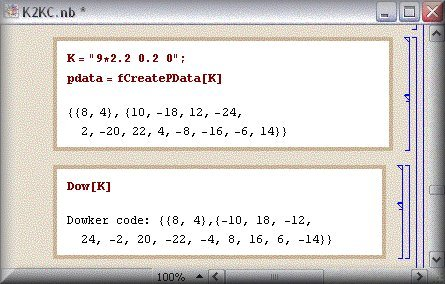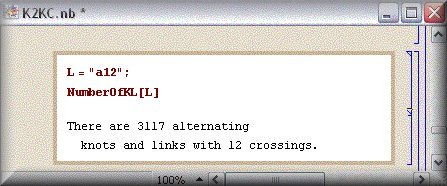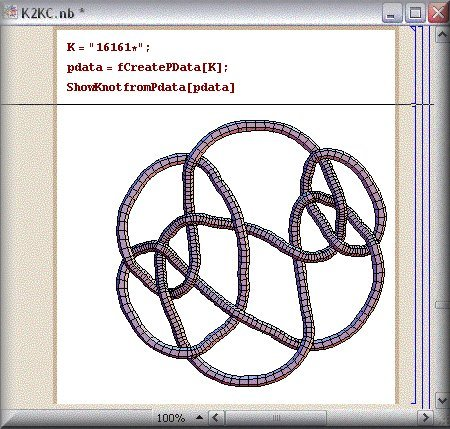Setup: LinKnot works in all versions of Mathematica (5.x, 6.x, 7.x, 8.x) 1) download the file LinKnot.zip; 2) extract it in the directory  C:\LinKnot Win-zip will automatically create new folder LinKnot 3) Depending on the version of Mathematica open the Mathematica-notebook file K2KL50.nb from the folder LinKnot in Mathematica 5.x,  K2KL60.nb in Mathematica 6.x, K2KL70.nb in Mathematica 7.x, or  K2KL80.nb in Mathematica 8.x and run the first line from the Introduction     SetDirectory["C:\\LinKnot"]      << LinKnots` LinKnot contains also some selected functions from the program Knot Theory. You can work in  LinKnot  with the complete program KnotTheory' , but the other Knot Theory functions are non visible. For the instructions how to use it together with the program KnotTheory', please visit the page  http://katlas.math.toronto.edu/wiki/Using_the_LinKnot_package  Short description: Mathematica-based program for Windows LinKnot  is the knot theory program that works with knots and links given in Conway notation. Conway symbols are input  used for creating Dowker codes, P-data ( the main input for K2K functions), or PD (planar diagrams, the main input for KnotTheory functions).LinKnot  contains the data bases of  knots and links with max. 12 crossings and virtual knots and links in Conway notation derived from knots and links with at most 8 crossings.Basic polyhedra with at most 20 crossings (e.g. , for 20 crossings there are 58782 polyhedra) are given in separate data bases. You can work with them in the same manner as with any other knot or link.NEXT